# The COUNTREG Procedure

### Prior Distributions

The PRIOR statement is used to specify the prior distribution of the model parameters. You must specify a list of parameters, a tilde (~), and then a distribution and its parameters. You can specify multiple PRIOR statements to define independent priors. Parameters that are associated with a regressor variable are referred to by the name of the corresponding regressor variable.

You can specify the special keyword _REGRESSORS to consider all the regressors of a model. If multiple prior statements affect the same parameter, the prior that is specified is used. For example, in a regression that uses three regressors (X1, X2, X3), the following statements imply that the prior on X1 is NORMAL(MEAN=0, VAR=1), the prior on X2 is GAMMA(SHAPE=3, SCALE=4), and the prior on X3 is UNIFORM(MIN=0, MAX=1):

...
prior _Regressors ~ uniform(min=0, max=1);
prior X1 X2 ~ gamma(shape=3, scale=4);
prior X1 ~ normal(mean=0, var=1);
...


If a parameter is not associated with a PRIOR statement or if some of the prior hyperparameters are missing, then the default choices shown in Table 11.3 are considered.

Table 11.3: Default Values for Prior Distributions

 PRIOR distribution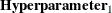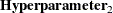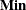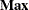Parameters Default Choice NORMAL MEAN=0 VAR=1E6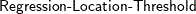IGAMMA SHAPE=2.000001 SCALE=1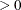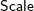GAMMA SHAPE=1 SCALE=1UNIFORMBETA SHAPE1=1 SHAPE2=1T LOCATION=0 DF=3For density specifications, see the section Standard Distributions.